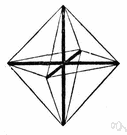# affine transformation

(redirected from Affine function)
Also found in: Thesaurus, Encyclopedia.
Related to Affine function: affine transformation
ThesaurusAntonymsRelated WordsSynonymsLegend:
 Noun 1affine transformation - (mathematics) a transformation that is a combination of single transformations such as translation or rotation or reflection on an axismath, mathematics, maths - a science (or group of related sciences) dealing with the logic of quantity and shape and arrangementtransformation - (mathematics) a function that changes the position or direction of the axes of a coordinate system
Based on WordNet 3.0, Farlex clipart collection. © 2003-2012 Princeton University, Farlex Inc.
References in periodicals archive ?
Factor [k.sub.1] together with [k.sub.2] make an affine function of reference stock level depending on market demand, given in the following form
Besides, this work has explained the process of normalization by homographic function and combination with an affine function by trying to sweep the present main measures in the literature with the aim of a presentation easier to understand.
Its border can be interpreted as the graph of a piecewise affine function [[omega].sub.[lambda]].
In , the systematical procedures for the analytical expression of explicit control laws of linear MPC via piecewise affine function are given, which saves the online computation time and memory requirements.
Consider that f is a non-constant [GAMMA]-linear affine function and let f'(b) be the left derivative of f at b.
ALPHA and MMALPHA use this syntax for specifying a multidimensional affine function in many different contexts (e.g., the projection function in a reduce expression).
Suppose that the function h is an affine function: h([x.sub.i]) = a + b[x.sub.i] where a is a nonnegative constant and b is a positive constant.
Using the mathematical induction, it can be proved that every affine function f: A [right arrow] R satisfies the equality
Moreover, the radius [[rho].sub.u] of F(z, u) is an affine function of u when u [member of] [-1,0]:
The function sin([phi]) is approximated by a piecewise affine function yielding a piecewise affine system with 5 regions as follows:

Site: Follow: Share:
Open / Close